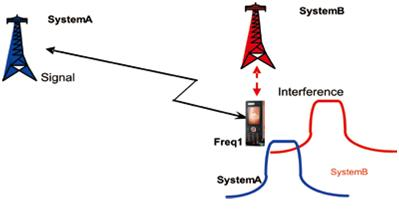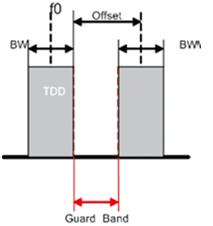TELETOPIX.ORG

Telecom Techniques Guide

LTE

# Adjacent Channel Interference Ratio for LTE

It is difficult to separate Adjacent Channel Leakage power Ratio and Adjacent channel selectivity because they coexist. therefore, Adjacent Channel Leakage power Ratio and Adjacent channel selectivity are usually considered together.

The result of the consideration is Adjacent Channel Interference Ratio (ACIR) and Adjacent Channel Interference Ratio is the ratio of the total power transmitted from a source (base station or UE) to the total interference power affecting a victim receiver, resulting from both transmitter and receiver imperfections. The formula is as follows:

ACIR =1/ ({1/ACLR} + {1/ACS})

The interference in the adjacent channel affects both the system coverage and system capacity. When near-far effect exists, interference from the adjacent channel greatly affects the system coverage, and may even cause the dead zone.As shown in the above figure, when the terminal in system A enters system B, the interference power of system B to system A is even greater than the useful power of system A. Therefore, the dead zone occurs, where the terminal cannot access the network.

In the uplink, the limiting design factor is the UE transmitter, which will dominate the uplink interference. The reason is that ACLRUE << ACSBS, which implies that uplink ACIR ≈ ACLRUE. Thus, in an uplink simulation, it is essentially the UE ACLR performance that is simulated.

In the downlink, the limiting design factor is the UE receiver, which will dominate the downlink interference. The reason is that ACSUE << ACLRBS, which implies that downlink ACIR ≈ ACSUE. A downlink simulation will thus essentially be a simulation of UE ACS performance.

OFFSET/BW/GB

Figure below is the relationship of the Offset (frequency offset value), BW (channel bandwidth) and Guard Band.